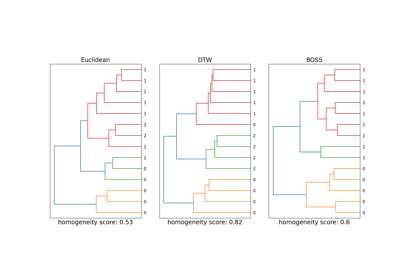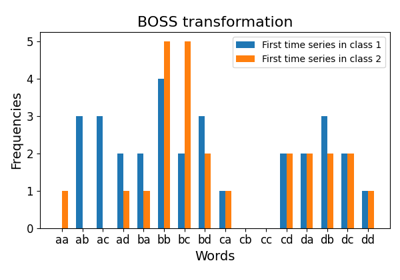# `pyts.transformation`.BOSS¶

class `pyts.transformation.``BOSS`(word_size=4, n_bins=4, strategy='quantile', window_size=10, window_step=1, anova=False, drop_sum=False, norm_mean=False, norm_std=False, numerosity_reduction=True, sparse=True, alphabet=None)[source]

Bag of Symbolic Fourier Approximation Symbols.

For each time series, subseries are extracted using a sliding window. Then the subseries are transformed into a word using the Symbolic Fourier Approximation (SFA) algorithm. For each time series, the words are grouped together and a histogram counting the occurences of each word is created.

Parameters: word_size : int (default = 4) Size of each word. n_bins : int (default = 4) The number of bins to produce. It must be between 2 and 26. strategy : str (default = ‘quantile’) Strategy used to define the widths of the bins: ‘uniform’: All bins in each sample have identical widths ‘quantile’: All bins in each sample have the same number of points ‘normal’: Bin edges are quantiles from a standard normal distribution ‘entropy’: Bin edges are computed using information gain window_size : int or float (default = 10) Size of the sliding window. If float, it represents the percentage of the size of each time series and must be between 0 and 1. The window size will be computed as `ceil(window_size * n_timestamps)`. window_step : int or float (default = 1) Step of the sliding window. If float, it represents the percentage of the size of each time series and must be between 0 and 1. The window size will be computed as `ceil(window_step * n_timestamps)`. anova : bool (default = False) If True, the Fourier coefficient selection is done via a one-way ANOVA test. If False, the first Fourier coefficients are selected. drop_sum : bool (default = False) If True, the first Fourier coefficient (i.e. the sum of the subseries) is dropped. Otherwise, it is kept. norm_mean : bool (default = False) If True, center each subseries before scaling. norm_std : bool (default = False) If True, scale each subseries to unit variance. numerosity_reduction : bool (default = True) If True, delete sample-wise all but one occurence of back to back identical occurences of the same words. sparse : bool (default = True) Return a sparse matrix if True, else return an array. alphabet : None, ‘ordinal’ or array-like, shape = (n_bins,) Alphabet to use. If None, the first n_bins letters of the Latin alphabet are used.

References

  P. Schäfer, “The BOSS is concerned with time series classification in the presence of noise”. Data Mining and Knowledge Discovery, 29(6), 1505-1530 (2015).

Examples

```>>> from pyts.datasets import load_gunpoint
>>> from pyts.transformation import BOSS
>>> X_train, X_test, _, _ = load_gunpoint(return_X_y=True)
>>> boss = BOSS(word_size=2, n_bins=2, sparse=False)
>>> boss.fit(X_train)
BOSS(...)
>>> sorted(boss.vocabulary_.values())
['aa', 'ab', 'ba', 'bb']
>>> boss.transform(X_test)
array(...)
```
Attributes: vocabulary_ : dict A mapping of feature indices to terms.

Methods

 `__init__`([word_size, n_bins, strategy, …]) Initialize self. `fit`(X[, y]) Fit the model according to the given training data. `fit_transform`(X[, y]) Fit the data then transform it. `get_params`([deep]) Get parameters for this estimator. `set_params`(**params) Set the parameters of this estimator. `transform`(X) Transform the provided data.
`__init__`(word_size=4, n_bins=4, strategy='quantile', window_size=10, window_step=1, anova=False, drop_sum=False, norm_mean=False, norm_std=False, numerosity_reduction=True, sparse=True, alphabet=None)[source]

Initialize self. See help(type(self)) for accurate signature.

`fit`(X, y=None)[source]

Fit the model according to the given training data.

Parameters: X : array-like, shape = (n_samples, n_timestamps) Training vector. y : None or array-like, shape = (n_samples,) Class labels for each data sample. self : object
`fit_transform`(X, y=None)[source]

Fit the data then transform it.

Parameters: X : array-like, shape = (n_samples, n_timestamps) Training vector. y : None or array-like, shape = (n_samples,) Class labels for each data sample. X_new : sparse matrix, shape = (n_samples, n_words) Document-term matrix.
`get_params`(deep=True)

Get parameters for this estimator.

Parameters: deep : bool, default=True If True, will return the parameters for this estimator and contained subobjects that are estimators. params : dict Parameter names mapped to their values.
`set_params`(**params)

Set the parameters of this estimator.

The method works on simple estimators as well as on nested objects (such as `Pipeline`). The latter have parameters of the form `<component>__<parameter>` so that it’s possible to update each component of a nested object.

Parameters: **params : dict Estimator parameters. self : estimator instance Estimator instance.
`transform`(X)[source]

Transform the provided data.

Parameters: X : array-like, shape = (n_samples, n_timestamps) Test samples. X_new : sparse matrix, shape = (n_samples, n_words) Document-term matrix.

## Examples using `pyts.transformation.BOSS`¶Time Series Clustering with DTW and BOSSBag-of-SFA Symbols (BOSS)